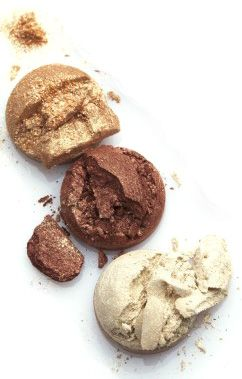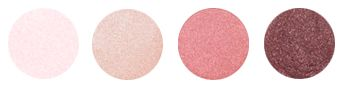G  L  A  M    I  T    U  P
​Micas, combined with subtle sparkles, give the eyes a glamorous WOW factor. The smooth formula is highly pigmented and very long wearing.
Use with Zinc Flat Tapered Shadow Brush.

LOOSE EYE SHIMMERSMOONSCAPE

GOLDSTONE

PATINA
GOLDEN
AMBER​
BITTERSWEET
BURNT
BROWN​
GLAZE
RED
VELVET​
ICE
BROWN​
TOURMALINE
BLACK
ORCHID​
SMOKED
PLUM​
GRANITE
CUPCAKE
BLUSHED
SUGARED ROSE
ATHENA
EMERALD
AZURE
INDIGO
NOIR
Behind the Brand___________Reviews
Home)))))))))))))Ordering)))))))))))))Shipping & Returns)))))))))))))Privacy)))))))))))))Terms)))))))))))))Ingredients)))))))))))))Contact